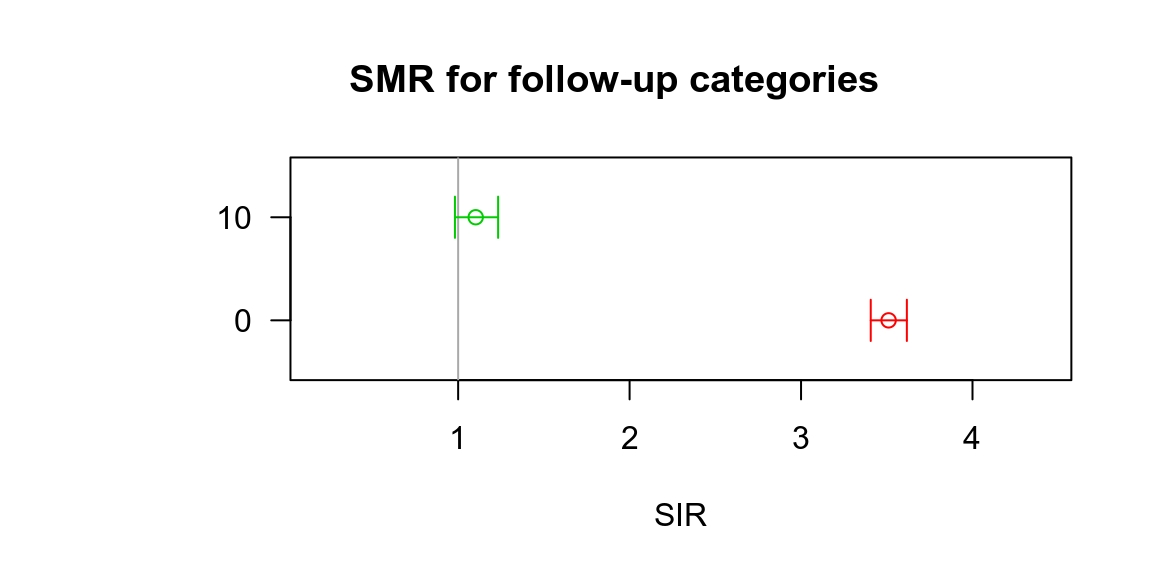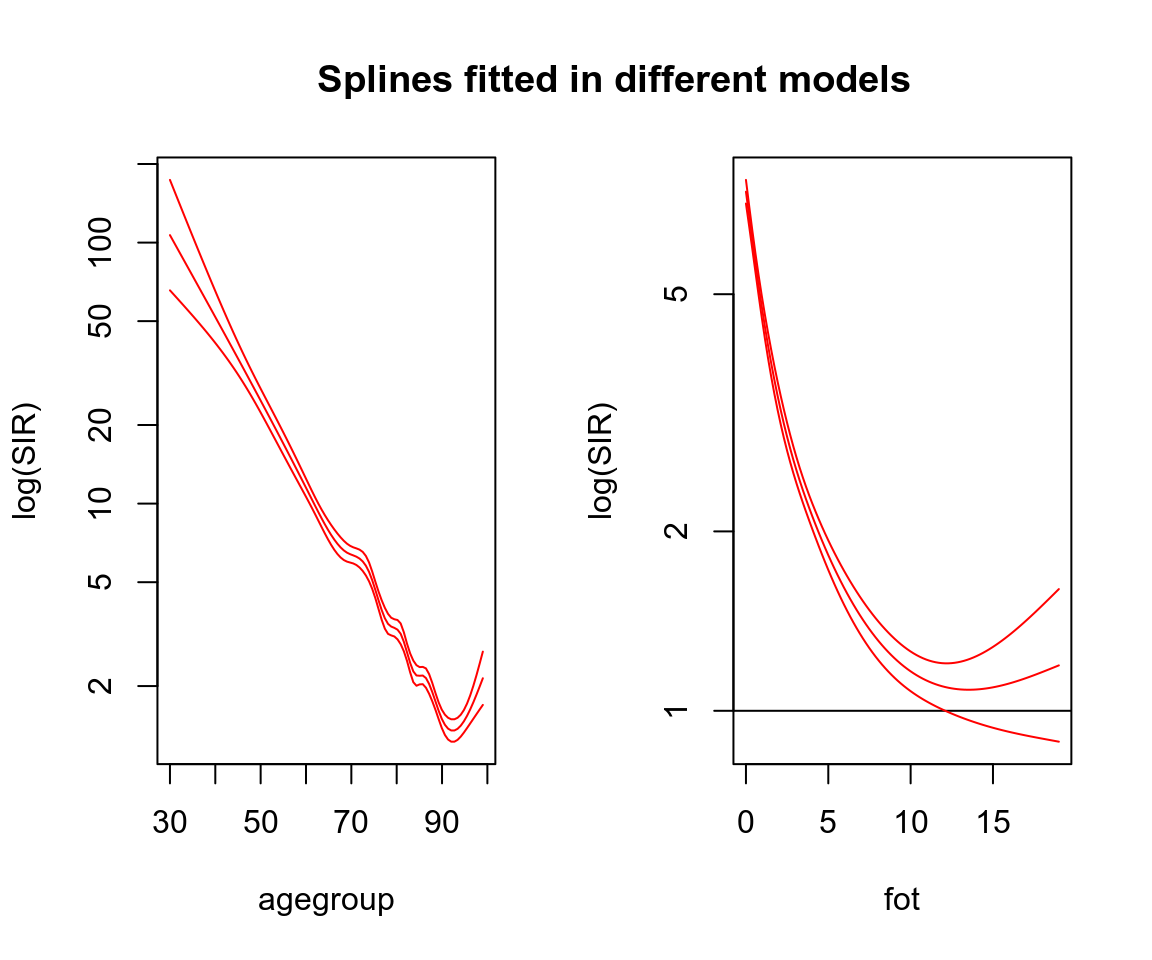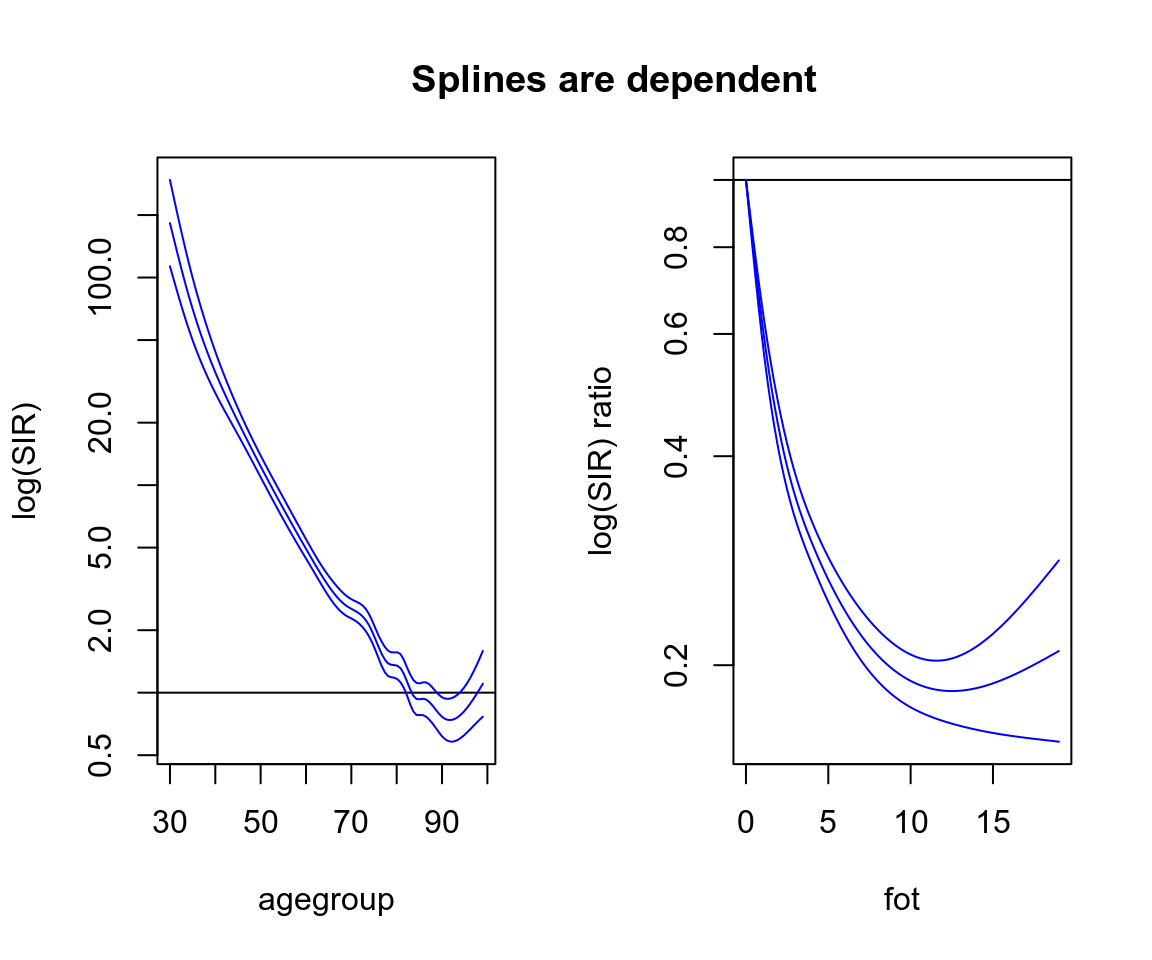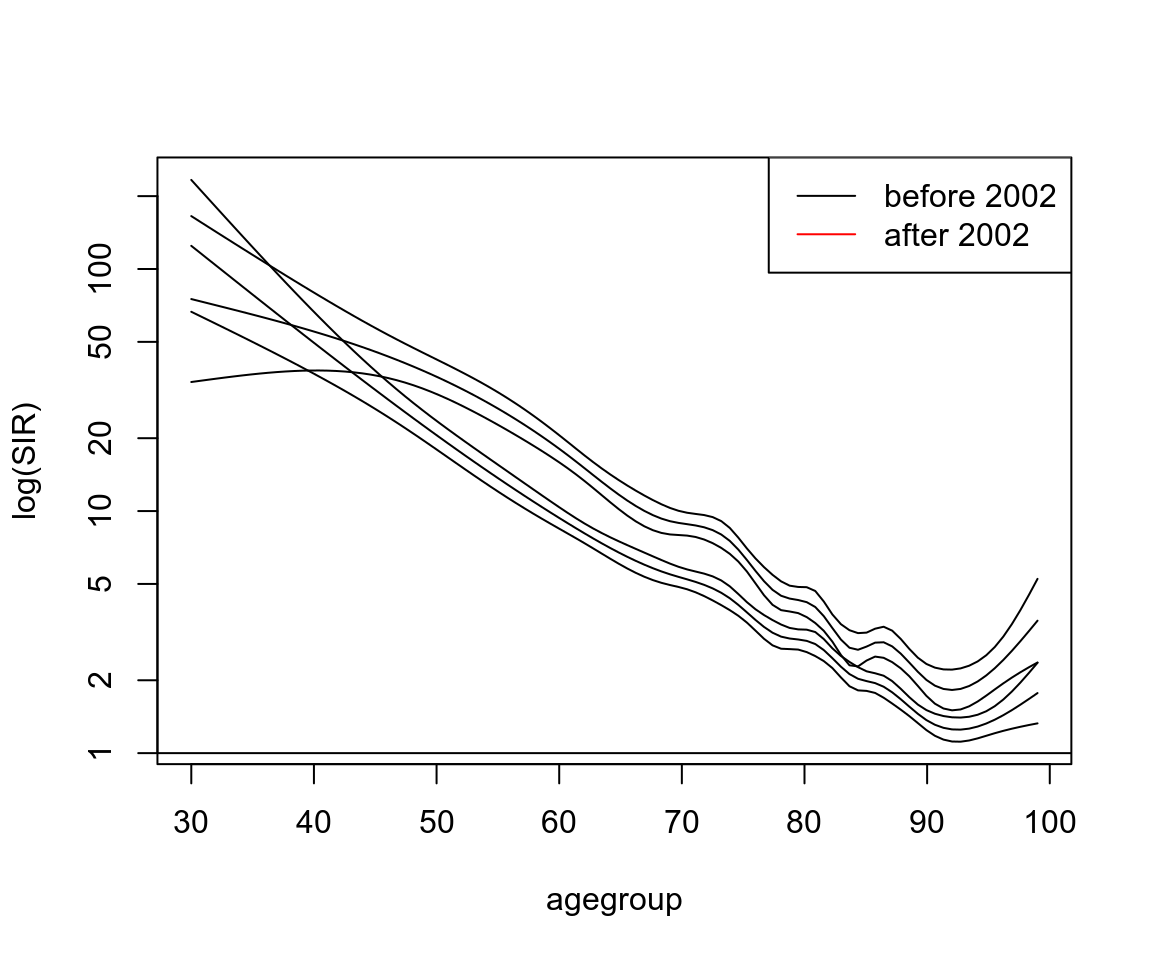library(popEpi)
library(Epi)
library(splines)

# Introduction

Standardized incidence ratio (SIR) or mortality ratio (SMR) is a ratio of observed and expected cases. Observed cases is the absolute number of cases in the cohort. The expected cases are derived by multiplying the cohort person-years with reference populations rate. The rate should be stratified or adjusted by confounding factors. Usually these are age group, gender, calendar period and possibly a cancer type or other confounding variable. Also a social economic status or area variable can be used.

In reference population the expected rate in strata $$j$$ is $$\lambda_j = d_j$$ / $$n_j$$, where $$d_j$$ is observed cases and $$n_j$$ is observed person years. Now the SIR can be written as a ratio $SIR = \frac{ \sum d_j }{\sum n_j \lambda_j} = \frac{D}{E}$ where $$D$$ is the observed cases in cohort population and $$E$$ is the expected number. Univariate confidence intervals are based on exact values of Poisson distribution and the formula for p-value is $\chi^2 = \frac{ (|O - E| -0.5)^2 }{E}.$ Modelled SIR is a Poisson regression model with log-link and cohorts person-years as a offset.

The homogeneity of SIR’s can be tested using a likelihood ratio test in Poisson modelled SIRs.

The same workflow applies for standardised mortality ratios.

# Splines

A continuous spline function can be fitted for time variables, e.g. age-group. Idea of the splines is to smooth the SMR estimates and do inference from the curve figure. This requires pre-defined knots/nodes that are used to fit the spline curve. Selecting the number of knots and knot places is a very subjective matter and there are three options to pass spline knots to function.

It’s good practice to try between different knot settings for realistic spline estimates. Overfitting might cause unintentional artefacts in the estimate and underfitting might smooth away interesting patterns.

The spline variable should be as continuous as possible, say from 18 to 100 time points. But when splitting time in too narrow intervals, random variation might occur in the expected or population rate values. Therefore it’s also possible to do two variables for age or period: first with wider intervals for standardisation and second with narrow intervals for the spline.

## Knots

There are three options to for assigning knots to the spline:

1. A vector of numbers of knots for each spline variable. Number of knots includes the boundary knots, so that the minimum number of knots is 2, which is a log linear association. The knots are placed automatically using the quantiles of observed cases.

2. A list of vectors of predefined knot places. Number of vectors needs to match the length of spline variables. And each vector has to have at least the minimum and maximum for boundary knots.

3. NULL will automatically finds the optimal number of knots based on AIC. Knots are placed according the quantiles of observed cases. This is usually a good place to start the fitting process.

Number of knots and knot places are always found in output.

# SMR

## Mortality: External cohort and popmort data

Estimate SMR of a simulated cohort of Finnish female rectal cancer patients, sire. Death rates for each age, period and sex is available in popmort dataset.

For more information about the dataset see help(popmort) and help(sire).

data(sire)
data(popmort)
c <- lexpand( sire, status = status, birth = bi_date, exit = ex_date, entry = dg_date,
breaks = list(per = 1950:2013, age = 1:100, fot = c(0,10,20,Inf)),
aggre = list(fot, agegroup = age, year = per, sex) )
## dropped 16 rows where entry == exit
se <- sir( coh.data = c, coh.obs = 'from0to2', coh.pyrs = 'pyrs',
ref.data = popmort, ref.rate = 'haz',
se
## SIR (adjusted by agegroup, year, sex) with 95% confidence intervals (profile)
## Test for homogeneity: p = 0.735
##
##  Total sir: 1.01 (0.95-1.06)
##  Total observed: 1490
##  Total expected: 1482.13
##  Total person-years: 39906
##
##
##    fot observed expected     pyrs  sir sir.lo sir.hi p_value
## 1:   0     1226  1214.54 34445.96 1.01   0.95   1.07  0.7423
## 2:  10      264   267.59  5459.96 0.99   0.87   1.11  0.8262

SMR’s for other causes is 1 for both follow-up intervals. Also the p-value suggest that there is no heterogeneity between SMR estimates (p=0.735).

The total mortality can be estimated by modifying the status argument. Now we want to account all deaths, i.e. status is 1 or 2.

c <- lexpand( sire, status = status %in% 1:2, birth = bi_date, exit = ex_date, entry = dg_date,
breaks = list(per = 1950:2013, age = 1:100, fot = c(0,10,20,Inf)),
aggre = list(fot, agegroup = age, year = per, sex) )
## dropped 16 rows where entry == exit
se <- sir( coh.data = c, coh.obs = 'from0to1', coh.pyrs = 'pyrs',
ref.data = popmort, ref.rate = 'haz',
se
## SIR (adjusted by agegroup, year, sex) with 95% confidence intervals (profile)
## Test for homogeneity: p < 0.001
##
##  Total sir: 3.08 (2.99-3.17)
##  Total observed: 4559
##  Total expected: 1482.13
##  Total person-years: 39906
##
##
##    fot observed expected     pyrs  sir sir.lo sir.hi p_value
## 1:   0     4264  1214.54 34445.96 3.51   3.41   3.62   0.000
## 2:  10      295   267.59  5459.96 1.10   0.98   1.23   0.094

Now the estimates for follow-up intervals seems to differ significantly, p = 0. Plotting SMR (S3-method for sir-object) is easily done using default plot-function.

plot(se, col = 2:3)
title('SMR for follow-up categories')## splines

Lets fit splines for the follow-up time and age group using two different options: the splines are fitted in different model and in same model, dependent.splines.

c <- lexpand( sire, status = status %in% 1:2, birth = bi_date, exit = ex_date, entry = dg_date,
breaks = list(per = 1950:2013, age = 1:100, fot = 0:50),
aggre = list(fot, agegroup = age, year = per, sex) )
## dropped 16 rows where entry == exit
sf <- sirspline( coh.data = c, coh.obs = 'from0to1', coh.pyrs = 'pyrs',
ref.data = popmort, ref.rate = 'haz',
spline = c('agegroup','fot'), dependent.splines=FALSE)

st <- sirspline( coh.data = c, coh.obs = 'from0to1', coh.pyrs = 'pyrs',
ref.data = popmort, ref.rate = 'haz',
spline = c('agegroup','fot'), dependent.splines = TRUE)

plot(sf, col=2, log=TRUE)
title('Splines fitted in different models')plot(st, col=4, log=TRUE)
title('Splines are dependent')In dependent spline the fot is the ratio with zero time as reference point. Reference points can be altered. Here age group profile is assumed to be same for every follow-up time. SMR is 0.2 times from 0 to 10 years of follow-up.

Splines can also be stratified using the print argument. For example we split the death time in two time periods and test if the age group splines are equal.

c$year.cat <- ifelse(c$year < 2002, 1, 2)
sy <- sirspline( coh.data = c, coh.obs = 'from0to1', coh.pyrs = 'pyrs',
ref.data = popmort, ref.rate = 'haz',
spline = c('agegroup'), print = 'year.cat')
plot(sy, log=TRUE)
legend('topright', c('before 2002','after 2002'), lty=1, col=c(1,2))For category before 2002 the SMR seems to be higher after the age of 50. Also the p-value (<0.0001) indicates that there is a difference in age group trends before and after year 2002. P-value is a likelihood ratio test that compares models where splines are fitted together and separately.

print(sy)
## agegroup: p < 0.001
## NA: p = NA
## NA: p = NA
##
##   levels colour
## 1      1  black
## 2      2    red